Find Paper, Faster
Example：10.1021/acsami.1c06204 or Chem. Rev., 2007, 107, 2411-2502
SMALL-BOUND ISOMORPHISMS OF FUNCTION SPACES
Journal of the Australian Mathematical Society  (IF0.8),  Pub Date : 2020-03-18, DOI: 10.1017/s1446788720000129
JAKUB RONDOŠ, JIŘÍ SPURNÝ

Let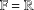$\mathbb{F}=\mathbb{R}$ or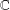$\mathbb{C}$ . For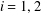$i=1,2$ , let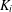$K_{i}$ be a locally compact (Hausdorff) topological space and let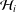${\mathcal{H}}_{i}$ be a closed subspace of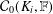${\mathcal{C}}_{0}(K_{i},\mathbb{F})$ such that each point of the Choquet boundary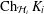$\operatorname{Ch}_{{\mathcal{H}}_{i}}K_{i}$ of${\mathcal{H}}_{i}$ is a weak peak point. We show that if there exists an isomorphism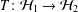$T:{\mathcal{H}}_{1}\rightarrow {\mathcal{H}}_{2}$ with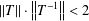$\left\Vert T\right\Vert \cdot \left\Vert T^{-1}\right\Vert <2$ , then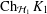$\operatorname{Ch}_{{\mathcal{H}}_{1}}K_{1}$ is homeomorphic to$\operatorname{Ch}_{{\mathcal{H}}_{2}}K_{2}$ . We then provide a one-sided version of this result. Finally we prove that under the assumption on weak peak points the Choquet boundaries have the same cardinality provided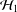${\mathcal{H}}_{1}$ is isomorphic to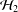${\mathcal{H}}_{2}$ .